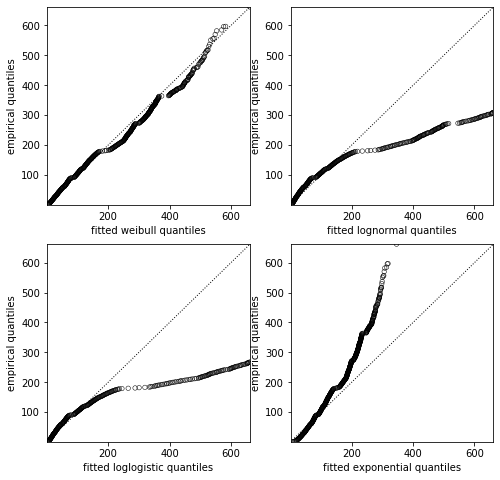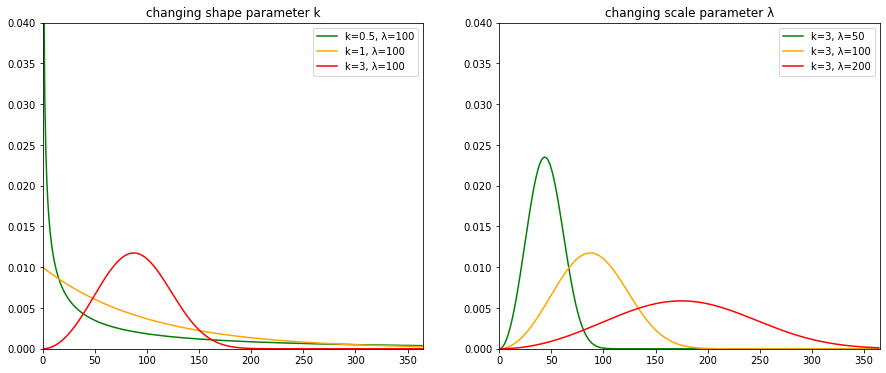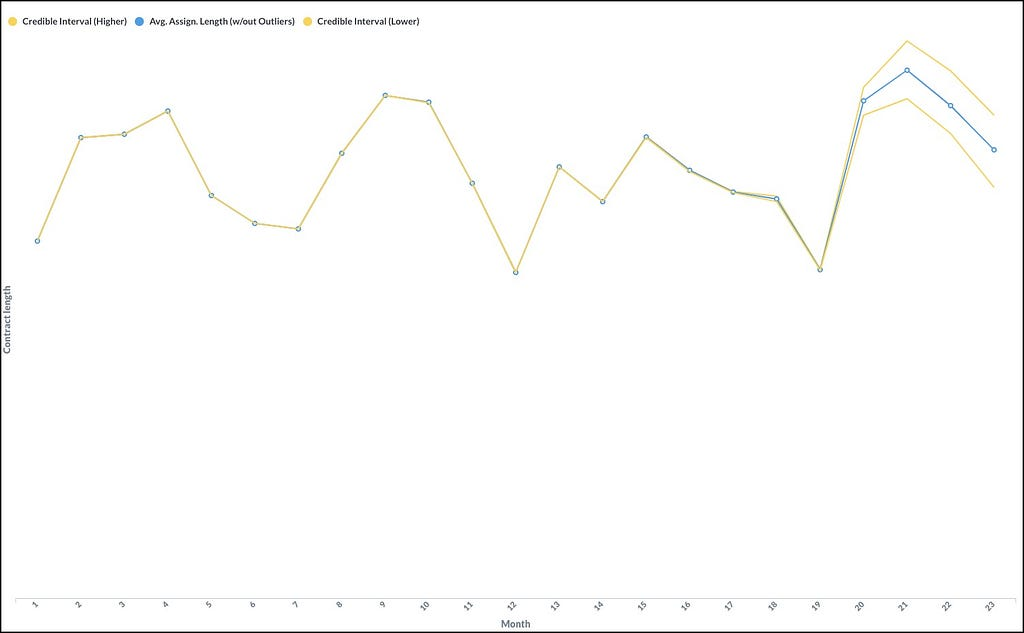# Predicting contract length with probabilistic programming

Problem overview Jobandtalent manages the lifecycle of thousands of jobs. From the requirements, to the recruitment, to the management of the contract, to the end of the contract. We even try to accompany the worker to their next opportunity. One of the key metrics in this industry is obviously the length of the contracts as […]

### Problem overview

Jobandtalent manages the lifecycle of thousands of jobs. From the requirements, to the recruitment, to the management of the contract, to the end of the contract. We even try to accompany the worker to their next opportunity.

One of the key metrics in this industry is obviously the length of the contracts as it is proportional to the revenue per contract, which is compared to the fixed and variable costs of managing it.

However, the length of the contracts is not an easy KPI to measure because we do not know in advance when a contract will end (they are often renewed many times, and they can also be shortened). Therefore if we were interested in the average length of the contracts that started this month, we would have to wait until all contracts have finished to finally know the true value (it can take a few years!). Can we make a good prediction instead?

The data team was tasked with tackling this challenge.

In the following sections, we detail our approach that mixes elements of survival analysis and Bayesian inference. In the end, we implemented an algorithm that provides an accurate prediction, continuously updating it as more information becomes available.

### The modeling process

#### Survival analysis

Survival analysis is the branch of statistics interested in modeling the time to an event. In this case, we are measuring the time between the creation (“birth”) and the end of a contract (“death”). As previously mentioned, the difficulty of the task is due to the fact that for all ongoing contracts, this data is missing. Those observations are defined as “censored” because at this point in time we don’t know yet when they will end.

The most important tool in survival analysis is the survival function. It is a curve along a time axis that displays, for a given time, the proportion of the population that is expected to be “alive”. This curve tells us all we need to know about the length of the “lives” of the population. In fact, it can easily be shown that this curve is simply 1-CDF(T), where T is the random variable representing the lifetime, and CDF(T) is its cumulative distribution function. Our problem can thus be solved if we could just estimate the survival function of T.

We choose to create a parametric model since we will need to predict the shape of the survival function well beyond the observed timeframe. However, this requires us to correctly specify a distribution.

The usual tool for selecting a distribution is a QQ-plot, but this is generally not appropriate for censored data. Thankfully, the lifelines python library contains routines to take censoring into account and still create Q-Q plots.The Q-Q plot compares two distributions (theoretical on the x-axis, observed on the y-axis). The more similar two distributions are, the closer the dots are to the diagonal. The Weibull distribution is by far the best match.

From the images above we can say that, among the distributions most used in survival analysis, the Weibull distribution fits the best. It is an approximation though, and we will have to take this into account when interpreting the results. Weibull distribution is characterized by two parameters, here called k and λ. λ is called the scale parameter because it controls how close to 0 the mass of the PDF (probability density function) is. k is called the shape parameter because it changes what the PDF looks like near 0.Weibull probability density function (PDF) for different values of k and λ.

#### Modeling over time

We would like to see the evolution over time of the length of the contract. It was decided that a month was the right granularity level, so we grouped the contracts by their month of creation. For each calendar month, we want to compute the average lifetime of the contracts that started during that month.

The easiest approach is to consider all of those populations independent. For each month, we can provide the lifelines API with the censored and uncensored lengths and it will return the values for λ and k that are most likely to generate those observations. However, we found that this approach created very unstable historical predictions and a very unreliable prediction for recent months. We needed a model that would include our knowledge that one month cannot be that different from the next. We also needed a model that would tell us how much confidence we can have in its predictions. Probabilistic Programming met our needs very well.

#### Probabilistic programming — the simple model

Probabilistic programming languages (or in our case, PyMC3, a Python library) simplify the creation of probabilistic models and the inference of the parameters of the model. In simple terms, we can specify our model:

`T ~ Weibull(λ, k)`

Then, we need to tell our model the durations of the finished contracts we have observed: finished contract durations (in days): [50, 110, 33,…]

In PyMC3, these two things can be done in a single line of code:

`pm.Weibull(‘T_observed’, alpha=k, beta=lambda_, observed=[50, 10, 33,…])`

It was very simple. Now, the idea is to let PyMC3 use its algorithm* to tell us what values λ and k can explain our observations. It will not return a single value, but a long list of samples that were taken from the joint posterior distribution of λ and k. We can just run: pm.sample()

This was the core of the model. It is missing a few parts though. First, we should specify a prior for the parameters λ and k. Second, we should also include unfinished contracts! They contain essential information since we would vastly underestimate the average length of the contracts if we ignored them. For a contract that is 40 days old, we know that the time to finish will be higher than 40. The probability of this happening is given by the survival function with parameters k and λ: sf(40, k, λ). For PyMC3 to take this into account we have to pass the log probability function to pm.Potential:

`pm.Potential(‘T_censored’, weibull_log_sf(censored_observations, alpha=k, beta=lambda_))`

Finally, we need to define all of the above within the context of a model (“with pm.Model():”). Here is a simple version of our model:

https://medium.com/media/70120668bde39d9cffc74999bf2be2cc/href

So far we have defined a model that can infer the Weibull distribution for a given period, in the form of a trace of samples for the pair (λ, k). Since we have samples for λ and k, we can easily compute the theoretical average for each pair and get a credible interval on the actual average. Next, we will see how we can make predictions of neighboring months dependent on each other.

#### Probabilistic programming — the time-dependent approach

Extending the model is relatively straightforward. We use a modeling trick (explained in more detail by Thomas Wiecki in his blog post). Essentially, we take the series of log(λ) (representing the scale parameter for Tᵢ, the lifetime of contracts of month i), and tell the model our prior assumption that they will follow a random walk with a certain standard deviation sigma. With this prior, the log(λ) can be positive or negative, but λ=exp(log(λᵢ)) will always be positive, which is required for Weibull distribution.

https://medium.com/media/b26871f1bdbd5db66c585d1945ffce49/href

In effect, it penalizes the posterior where λᵢ is very different from λᵢ₊₁. “Very different” depends on sigma, which is itself a parameter learned from the data (with its own prior).The blue line shows the forecasted avg. assignment length while the yellow lines represent the credible interval. Note that the oldest estimations are more stable since the majority of contracts had already ended at the time of the forecasting. The y-axis has been hidden to protect confidential data.

#### Computing any KPI

From the previous section, we get estimates for λᵢ and k. We can use them directly to compute the “underlying” mean lifetime of the contracts of each month. Alternatively, we can use them to simulate only the lifetimes of the contracts that are still ongoing (sampling from the conditional distribution P(T|T>time_until_today)). By averaging over many of those simulations we can get an estimate of the average length we will actually observe.

Since our approach is based on creating realistic simulations, we can generalize it to compute not only the expected average length but any KPI based on the length of the contracts (such as the average length without outliers).

### Project evolution and conclusion

We have worked in close collaboration with the Strategy team, one of the main stakeholders for this metric. We had to make sure we covered their requirements and that they understood our approach.

• The first step was to understand how they had been computing this metric previously, and how they use it.
• Secondly, we set out to create the most naive predictor possible. It allowed us to quickly get acquainted with the data and understand the challenges. This provided us with a first baseline.
• Finally, we started iterating over more and more complex models, taking care to only add complexity where needed and to maintain the feedback loop with the Strategy team through weekly meetings. These iterations resulted in the model described above.

The flexibility of our approach continued to pay off while we quickly iterated to create the KPIs that would fit their needs (actionable and unbiased by outliers). The Bayesian approach offers many advantages in this regard:

• Hypotheses are clearly stated in the code that mimics the generation process of the data.
• It generates realistic scenarios, based on which any KPI can easily be computed..
• It provides uncertainty estimates for free.
• It is a clear and principled approach, no black box required.
• Parts of the model can easily be reused. For example, we created a variation of the model that differentiates contracts by the reason they are ending. It allowed us to predict changes to the contract length under different churn scenarios.

*to read more about MCMC, a technique to get samples from the posterior distribution, a good starting point is: http://elevanth.org/blog/2017/11/28/build-a-better-markov-chain/

### We are hiring!

https://medium.com/media/7171964a0e0f5cd8313526de1e33393f/href

If you want to know more about it’s like work at Jobandtalent you can read the first impressions of some of our teammates in this blog post or visit our twitter.Predicting contract length with probabilistic programming was originally published in Jobandtalent Engineering on Medium, where people are continuing the conversation by highlighting and responding to this story.

Source: Jobandtalent